Mode

(diff) ← Older revision | Latest revision (diff) | Newer revision → (diff)
One of the numerical characteristics of the probability distribution of a random variable. For a random variable with density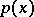(cf. Density of a probability distribution), a mode is any pointwhereis maximal. A mode is also defined for discrete distributions: If the values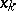of a random variablewith distribution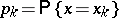are arranged in increasing order, then a point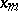is called a mode if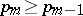and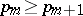.
Distributions with one, two or more modes are called, respectively, unimodal (one-peaked or single-peaked), bimodal or multimodal. The most important in probability theory and mathematical statistics are the unimodal distributions (cf. Unimodal distribution). Along with the mathematical expectation and the median (in statistics) the mode acts as a measure of location of the values of a random variable. For distributions which are unimodal and symmetric with respect to some point, the mode is equal toand to the median and to the mathematical expectation, if the latter exists.